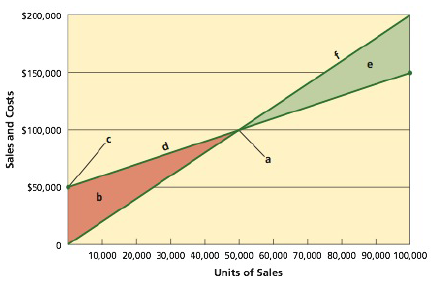Chapter 21, Problem 21.19EX

Chapter
Section
Textbook Problem

Break-even chartName the following chart and identify the items represented by the letters (a) through (f):To determine

Break-even (Cost-Volume-Profit) chart: It is a graphical representation of the relationship between the sales, costs, and the related profit or loss at various levels of units sold. In other words, it shows the effect of the changes in the cost and the sales volume on the operating income of the company.

To name and identify: the items represented by the letters (a) through (f) in the given chart.

Explanation

(a)

The break-even point is the intersection point where the total sales line and total cost line meet. A vertical dotted line is drawn downward from the intersection point to reach at the break-even sales (units). And, a horizontal line is drawn to the left towards the vertical axis to reach at the break-even sales (dollars).

(b)

The operating loss area is indicated towards the left of the intersection point. The area is indicated in red color. It is the area where the total cost line exceeds the total sales line.

(c)

It is the total fixed cost (\$50,000) point on the vertical axis from where the total cost line starts is drawn upward towards the maximum relevant range of level of sales units (100,000 units) on the horizontal axis.

(d)

The total cost is the sum of the fixed cost and variable cost. The total costs are shown in dollars on the vertical axis...

Still sussing out bartleby?

Check out a sample textbook solution.

See a sample solution

The Solution to Your Study Problems

Bartleby provides explanations to thousands of textbook problems written by our experts, many with advanced degrees!

Get Started

Find more solutions based on key concepts